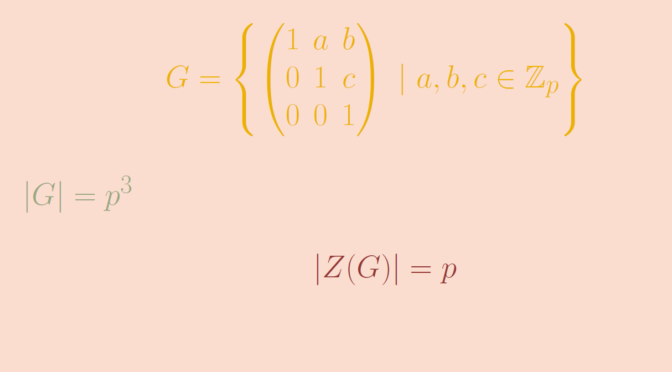# A nonabelian $$p$$-group

Consider a prime number $$p$$ and a finite p-group $$G$$, i.e. a group of order $$p^n$$ with $$n \ge 1$$.

If $$n=1$$ the group $$G$$ is cyclic hence abelian.

For $$n=2$$, $$G$$ is also abelian. This is a consequence of the fact that the center $$Z(G)$$ of a $$p$$-group is non-trivial. Indeed if $$\vert Z(G) \vert =p^2$$ then $$G=Z(G)$$ is abelian. We can’t have $$\vert Z(G) \vert =p$$. If that would be the case, the order of $$H=G / Z(G)$$ would be equal to $$p$$ and $$H$$ would be cyclic, generated by an element $$h$$. For any two elements $$g_1,g_2 \in G$$, we would be able to write $$g_1=h^{n_1} z_1$$ and $$g_2=h^{n_1} z_1$$ with $$z_1,z_2 \in Z(G)$$. Hence $g_1 g_2 = h^{n_1} z_1 h^{n_2} z_2=h^{n_1 + n_2} z_1 z_2= h^{n_2} z_2 h^{n_1} z_1=g_2 g_1,$ proving that $$g_1,g_2$$ commutes in contradiction with $$\vert Z(G) \vert < \vert G \vert$$. However, all $$p$$-groups are not abelian. For example the unitriangular matrix group $U(3,\mathbb Z_p) = \left\{ \begin{pmatrix} 1 & a & b\\ 0 & 1 & c\\ 0 & 0 & 1\end{pmatrix} \ | \ a,b ,c \in \mathbb Z_p \right\}$ is a $$p$$-group of order $$p^3$$. Its center $$Z(U(3,\mathbb Z_p))$$ is $Z(U(3,\mathbb Z_p)) = \left\{ \begin{pmatrix} 1 & 0 & b\\ 0 & 1 & 0\\ 0 & 0 & 1\end{pmatrix} \ | \ b \in \mathbb Z_p \right\},$ which is of order $$p$$. Therefore $$U(3,\mathbb Z_p)$$ is not abelian.